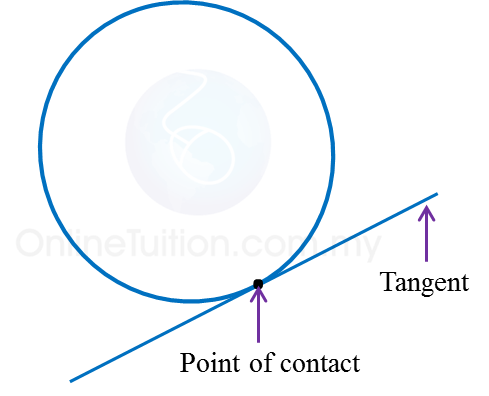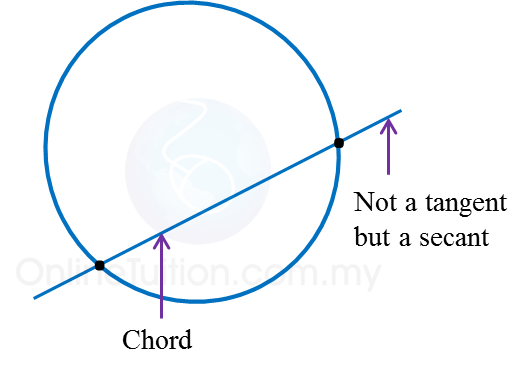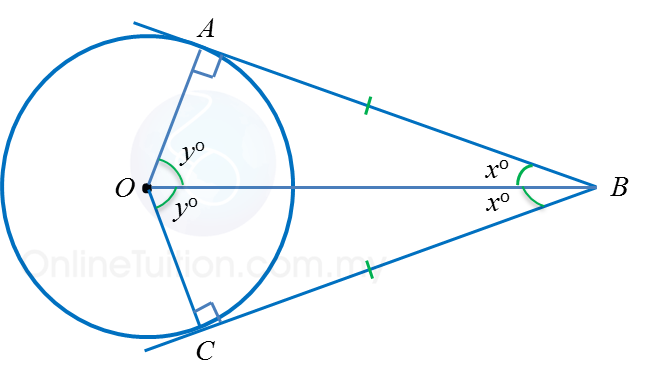# 6.3.1 Tangents to a Circle

6.3.1 Tangents to a Circle

1. A tangent to a circle is a straight line that touches the circle at only one point and the point is called the point of contact.2. If a straight line cuts a circle at two distinct points, it is called a secant. The chord is part of the secant in a circle.3. Tangent to a circle is perpendicular to the radius of the circle that passes through the point of contact.If ABC is the tangent to the circle at B, then $\angle$ ABO = $\angle$ CBO = 90o

Properties of Two Tangents to a Circle from an External PointIn the diagram, BA and BC are two tangents from an external point B. The properties of the tangents are as follows.

 (a) BA = BC (b) $\angle$ ABO = $\angle$ CBO = xo (c) $\angle$ AOB = $\angle$ COB = yo (d) ∠ OAB = $\angle$ OCB = 90o (e) $\angle$ AOC + $\angle$ ABC = 180o (f) ∆ AOB and ∆ COB are congruent